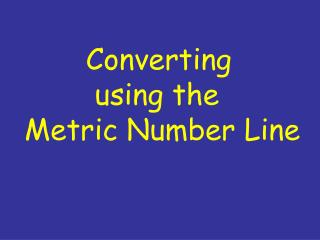Download PresentationConverting using the Metric Number Line

# Converting using the Metric Number Line - PowerPoint PPT PresentationDownload Presentation## Converting using the Metric Number Line

- - - - - - - - - - - - - - - - - - - - - - - - - - - E N D - - - - - - - - - - - - - - - - - - - - - - - - - - -
##### Presentation Transcript

1. Converting using the Metric Number Line

2. The Number Line T G M k h da d c m μ n p P R E F I X H E C T O C E N T I M I L L I M I C R O G I G A M E G A K I L O D E K A D E C I N A N O P I C O T E R A 3 3 3 1 1 1 1 1 1 3 3 3 Base Unit (m,L,g)

3. Rules for Using the Number Line 1. Find the pre-fix of the unit that is given in the problem. Locate this on the number line. This is the first letter in the unit. T G M k h da d c m μ n p 3 3 3 1 1 1 1 1 1 3 3 3 Example: 567.3 km = _____ cm

4. 2. Find the pre-fix that of the conversion you want to find on the number line. T G M k h da d c m μ n p 3 3 3 1 1 1 1 1 1 3 3 3 Example: 567.3 km = _____ cm

5. 3. Count the spaces using the numbers between the two pre-fixes. T G M k h da d c m μ n p 3 3 3 1 1 1 1 1 1 3 3 3 Example: 567.3 km = _____ cm Number of Spaces = ____

6. 4. Move the decimal point in the same direction as you are going on the number line. Example: 567.3 km = _____ cm 567.3

7. Base Unit (m,L,g) T G M k h da d c m μ n p 3 3 3 1 1 1 1 1 1 3 3 3 a. 75 cm = _____ m b. 1253 mL = _____ kL c. 5 ng = _____ cg d. 34 Tm = _____ hm